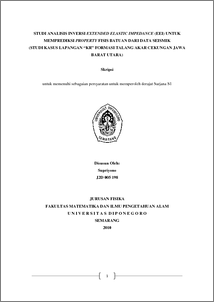# STUDI ANALISIS INVERSI EXTENDED ELASTIC IMPEDANCE (EEI) UNTUK MEMPREDIKSI PROPERTY FISIS BATUAN DARI DATA SEISMIK (STUDI KASUS LAPANGAN “KR” FORMASI TALANG AKAR CEKUNGAN JAWA BARAT UTARA)

Supriyono, Supriyono (2010) STUDI ANALISIS INVERSI EXTENDED ELASTIC IMPEDANCE (EEI) UNTUK MEMPREDIKSI PROPERTY FISIS BATUAN DARI DATA SEISMIK (STUDI KASUS LAPANGAN “KR” FORMASI TALANG AKAR CEKUNGAN JAWA BARAT UTARA). Undergraduate thesis, Department of Physics, Diponegoro University.Preview
PDF
66Kb

## Abstract

Extended Elastic Impedance (EEI) inversion method can be used to predict the physical properties of rock such as Gamma Ray, Vshale, Density, P-wave velocity, Poisson Ratio, Lambda*Rho,and Resistivity of seismic data. EEI in this study was conducted to identify and map the distribution of fluid hydrocarbons in the layer of the ST-06 Talang Akar formation with sandstone lithology. The Amplitude Variation with Offset (AVO) inversion result such as Intercept and Gradient attribute is used as input to make EEI reflectivity. The analysis of AVO graphics and angle stack (near angle stack and far angle stack) attributes to determine amplitude response to offset is used to determine AVO anomaly class. Furthermore, it is conducted crossplot analysis from the rock physical parameters by the existed data log. The used EEI inversion method is sparse spike zero phase. The result of EEI inversion is EEI(55) equivalent with Gamma Ray; EEI(49) equivalent with Vshale; EEI(11) equivalent with Density; EEI(-3) equivalent with P-wave velocity; EEI(44) equivalent with Poisson Ratio; EEI(21) equivalent with Lambda*Rho; and EEI(73) equivalent with Resistivity. The analysis result of slice horizon ST-06 map and cross section EEI inline 1746, the value EEI(55) is 8600-9400 (m/s)*(gr/cc) equivalent with Gamma Ray; EEI(49) value is 8800-9400(m/s)*(gr/cc) equivalent with Vshale; EEI(11) value is 8600-9400 (m/s)*(gr/cc) equivalent with Density; EEI(-3) value is 10200-10600 (m/s)*(gr/cc) equivalent with P-wave velocity; EEI(44) value is 8600-9200 (m/s)*(gr/cc) equivalent with Poisson Ratio; EEI(21) value is 9000-9400 (m/s)*(gr/cc) equivalent with Lambda*Rho; and EEI(73) value is 11500-13000 (m/s)*(gr/cc) equivalent with Resistivity.

Item Type: Thesis (Undergraduate) Inversion, AVO, Extended Elastic Impedance (EEI), Seismic Data Q Science > QC Physics Faculty of Science and Mathematics > Department of Physics 19873 Ms. Wien Admin 29 Nov 2010 11:29 29 Nov 2010 11:29

Repository Staff Only: item control page